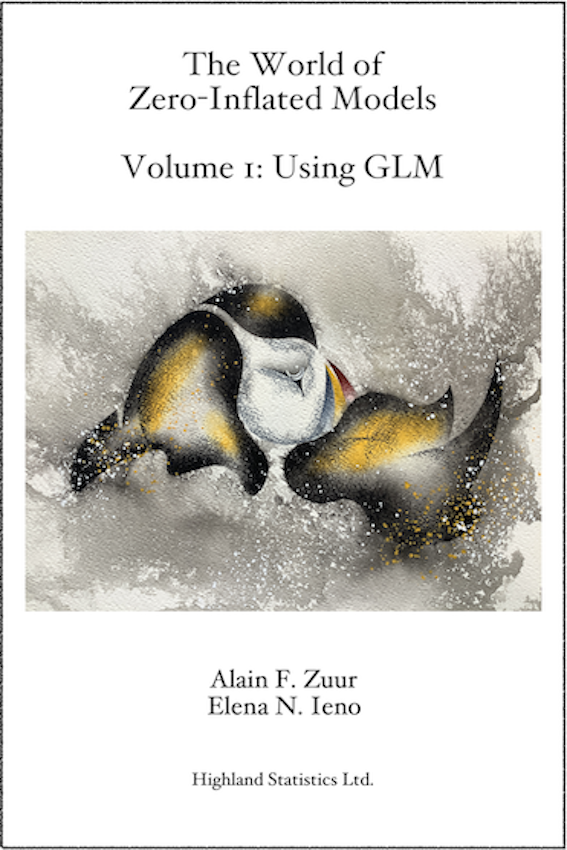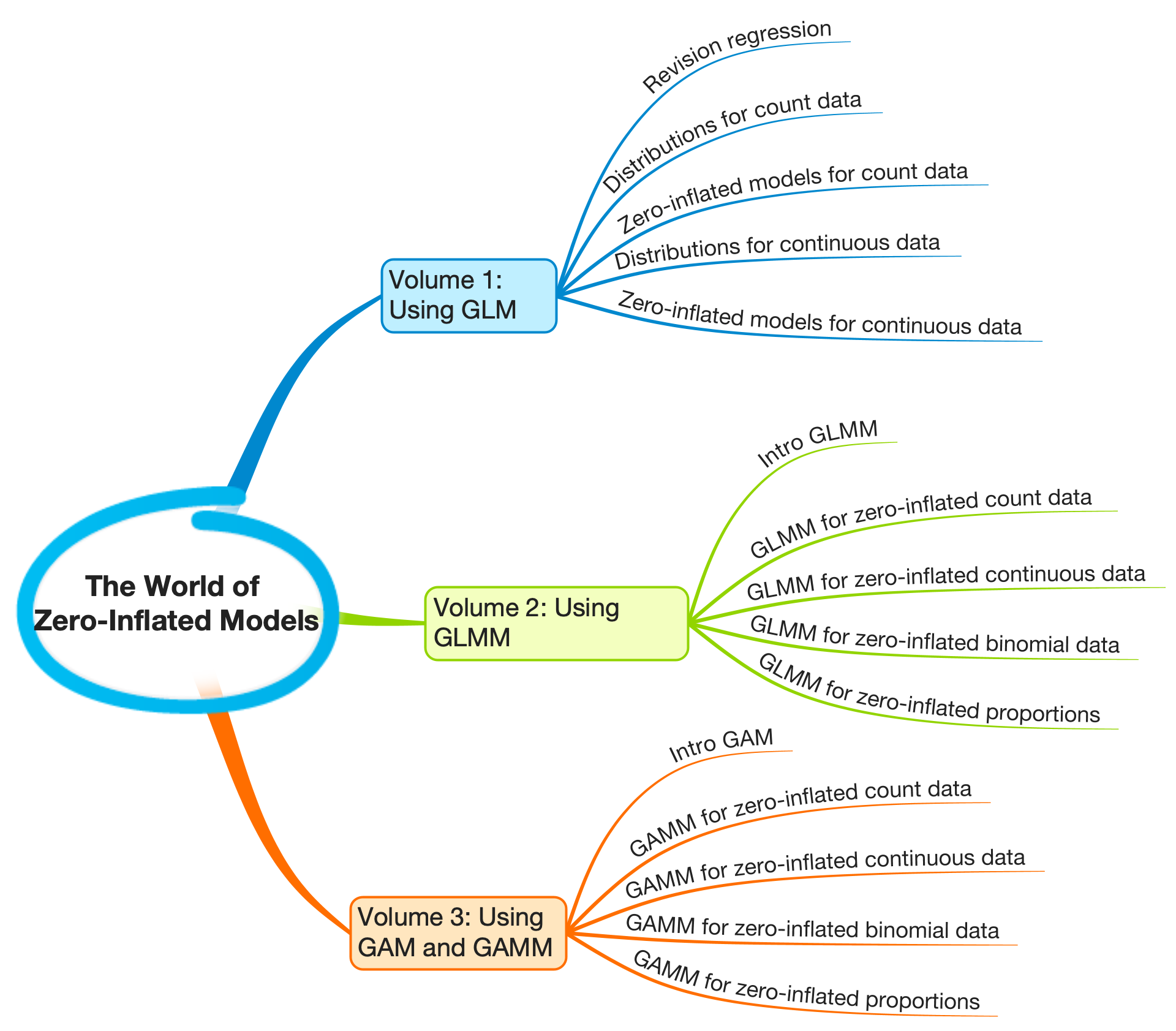#### Zuur AF and Ieno EN

Volume I was published in December 2021. Volume II will be available in September 2023. Volume III is scheduled for 2024.

When you buy this book or E-book, you will get free electronic access to 'Zero Inflated Models and Generalized Linear Mixed Models with R' by Zuur, Savaliev, Ieno (2012).

The world of zero-inflated models is large and complex. The first layer of complexity is the type of data. In Volumes 1 to 3 we will analyse count data, continuous data, proportional data, density data, etc.The second layer of complexity is that, for each of these data types, we have multiple options for choosing a statistical distribution. For count data we will discuss the Poisson, negative binomial (NB), generalised Poisson (GP) and Conway–Maxwell–Poisson (CMP) distributions. For continuous data we will apply the Tweedie distribution and the zero-altered Gamma (ZAG) approach. For proportional data we will use the binomial and the beta distributions.

The third layer of complexity is pseudo-replication. We may have multiple observations from the same site, animal, person, etc. This brings us within the world of linear mixed-effects models and generalised linear mixed models (GLMMs). This is the topic of Volume 2.

The fourth layer of complexity is that some covariates may have a non-linear effect, which may require generalised additive models (GAMs). If your data sets requires zero-inflated GAM or zero-inflated generalized additive mixed models (GAMMs), then Volume 3 of this series will help you analyse your data. If on top of this, you also have spatial, temporal, or spatial-temporal dependency, then there is no escape from R-INLA. Note that with spatial dependency, we assume that you have 50+ spatial locations. And temporal dependency becomes relevant if you have 15+ measurements over time. Zero-inflated spatial and spatial-temporal models are discussed in Zuur and Ieno (2018)

Volumes 1, 2 and 3

The text before you is Volume 1 of The World of Zero-Inflated Models. We will discuss models for count data and continuous data with an excessive number of zeros. All models are extensions of generalised linear models (GLMs). In Volume 2, we will analyse count data, continuous data and proportional data using GLMMs. Hence, we will extend the models from Volume 1 with random intercepts and random slopes. In Volume 3, we will extend the models from Volumes 1 and 2 towards GAMs and GAMMs. A schematic overview of Volumes 1 – 3 is presented in Figure 1.1.

Volume 1 can be read as a stand-alone. Volume 2 assumes that you have read Volume 1, and Volume 3 is a continuation of Volume 2.Outline of Volume

In Chapter 2 we revise data exploration and multiple linear regression using red knot data. Stable isotope ratios of nitrogen in animal tissues are modelled as a function of 3 covariates. This chapter serves as a blueprint for all other chapters in the sense that it shows the general outline of a statistical analysis.

Chapter 3 starts with a revision of the Poisson distribution and the Poisson GLM for the analysis of count data. We use a small puﬀin data set. We also introduce the NB GLM and two relatively unknown, but useful, members of the family, namely the GP GLM and the CMP GLM. Surprisingly, the latter two models tend to perform better than the NB GLM in the case of overdispersion. The latter two can also be used to deal with underdispersion. Most models are fitted with the glmmTMB package in R. Model validation tools are explained, and the concept of simulating data from a model (to verify whether it complies with all assumptions of the model) is introduced. We first do the simulation steps ourselves, then quickly migrate to the DHARMa package, which is rapidly gaining popularity.

In Chapter 4 we introduce zero-inflated models for count data, and these are executed with the glmmTMB package. We start with a basic introduction using simulated data, and discuss zero-inflated Poisson (ZIP), zero-inflated NB (ZINB), zero-inflated generalised Poisson (ZIGP) and zero-inflated CMP (ZICMP) models. We then apply them all on the puﬀin data set.

In Chapter 5 we analyse data on parasites in Brazilian sandperch. Such data nearly always bring you within zero-inflation territory. Now that we are familiar with Poisson, NB, GP, CMP models, and their zero-inflated cousins, it is time to learn how we can manoeuvre among them. How do we decide to apply an NB GLM or a ZIP model? In this chapter, we will keep the binary part of the model simple.

Chapter 6 is about ZIGP models. Data on mistletoe tree infections are used. The ZIGP models contain covariates in both the count and binary parts of the model.

Hurdle models for count data are discussed in Chapter 7 using dolphin sighting data. In a hurdle model we perform 2 analyses. First, the sighting abundances are converted into absence/presence data, and a Bernoulli GLM is applied. Then the zero counts are set to NA (or dropped), and a truncated Poisson (or NB) GLM is applied. In the third step, the two components are combined to calculate the expected values of the hurdle model. Chapter 7 is relatively long as it contains many topics that may be relevant: Bernoulli GLM, quasi-separation, truncated Poisson and NB distributions, and zero-altered Poisson (ZAP) and zero-altered NB (ZANB) models.

In the last 2 chapters of this volume, we discuss models for the analysis of continuous data with an excessive number of zeros. Biomass of lobsters are analysed using Tweedie GLMs in Chapter 8, and a ZAG model is applied on the same data in Chapter 9. The ZAG is a hurdle model for continuous data. Our recommendation is to opt for the Tweedie GLM approach.

#### Data and R code VOLUME I

All data is freely available. All the R code is provided as well, except that a password is needed to open the zip files. The password is given in the Preface of each book.

All data for Volume I: AllDataZIPBookVol1.zip

All R code for Volume I: AllRcodeZIPBookVol1.zip. We used the R-Markdown files from the book and removed all text except for the blocks with R code and the section headers (so you can see where you are in a chapter).

• Unzip the file using the password that is in the Preface of the book. The data sets are also in this file.
• Click on one of the Rmd files. It will open in RStudio.
• Change the working directory in the setwd() function.
• Option 1 to execute the code: Click on the knitting symbol in RStudio (the blue ball with the needle through it).
• Option 2 to execute the code: Click on the green triangle of each so-called chunk.
• Option 3 to execute the code: If you do not fancy RMarkdown code, then you can also copy-paste the R code within the chunks into an ordinary R file (you can also extract the R code from an RMarkdown document automatically, see this link).
• Send us an email in case of errors.